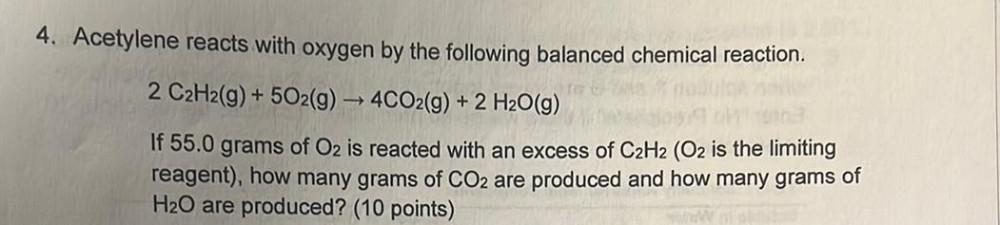Question:

# 4. Acetylene reacts with oxygen by the following balanced chemical reaction. 2 C2H2(g) + 502(g) → 4CO2(g) + 2 H2O(g) If 55.0 gra4. Acetylene reacts with oxygen by the following balanced chemical reaction. 2 C2H2(g) + 502(g) → 4CO2(g) + 2 H2O(g) If 55.0 grams of Oz is reacted with an excess of C2H2 (O2 is the limiting reagent), how many grams of CO2 are produced and how many grams of H20 are produced? (10 points)